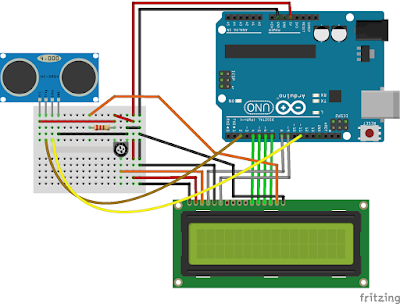### Ultrasonic Tape Measure with HC-SR04 Sensor

Easy to build Arduino powered ultrasonic distance calculator with data recording and LCD display.

The wide availability and low price of microcontrollers makes it possible to update older analog designs to create reliable digital devices that are better and have more features. This time, using an ultrasonic sensor controlled by a microcontroller you can build an electronic distance calculator that can be used as an alternative to a tape measure for distances between 2 and 400 cm. I chose an ATmega development board (Arduino compatible) with an alphanumeric LCD to calculate and display data from the ultrasonic sensor.

The measuring device can be built entirely from ready made breakout boards and it can be powered from a 9V battery. With ATmega as its core, the device can have many features. The software supports current measurement hold and EEPROM storage. Stored measurement can be then displayed at a push of a button. The mode of operation is continuous. Measurements are performed each at 100 ms intervals and displayed on the LCD as long as the device is powered. You can pause the measurement by pressing the button assigned to HOLD function.

The device in the below photo can be built inside a case as long as the sensor's transmitter and receiver are passed through holes in the case. You will also need some holes for the display and buttons.

The hardware is made of an Arduino compatible board on top of which is the LCD keypad shield. All it needs is the sensor which requires two signal pins. The Arduino board can be powered from 5V via USB port or from 9V battery using the DC jack. The sensor I used is the popular HC-SR04, which is an integrated device with microcontroller. When this microcontroller receives a trigger pin, it activates the ultrasonic transmitter which sends a 40kHz pulse into the air. The signal is reflected over surfaces and returns to the device, where it is captured by the receiver. Received signal is amplified and filtered before being fed to the microcontroller which computes the time required for the signal to arrive at receiver. The microcontroller outputs a pulse with a duration equal to the time the ultrasonic wave traveled through air. Arduino captures this pulse and measures its width. Assuming the speed of sound through air is 340 meters per second, we can calculated the distance. According to the datasheet, the accuracy of the sensor can reach 3mm.

Unlike other Arduino shields and breakout boards, this one is very simple to use and it doesn't need a specific library. Simply send a 10 us pulse to the sensor MCU, then measure the pulse width of the echo signal. Here comes handy the pulseIn() function, whose purpose is exactly this. A library is however needed for the LCD. And you need to write your own code for the analog keypad.

I came up with a rather simple sketch that displays the measured distance on LCD. If you press the SELECT button you can toggle HOLD mode. UP button stores current measurement into EEPROM memory of ATmega MCU and DOWN button displays the value from memory (RECALL). The software can be improved to store multiple measurements for example. I also provided a macro definition of an OFFSET which is added to the displayed distance. This is useful in the following scenario: by default, the sensor measures distance from itself to an object. But you may want to measure the distance from the opposite edge of the case or from a marking on the device case. Here you should set a negative value for OFFSET.
#include <LiquidCrystal.h>
#include <EEPROM.h>

#define OFFSET 0

#define btnRIGHT  0
#define btnUP     1
#define btnDOWN   2
#define btnLEFT   3
#define btnSELECT 4
#define btnNONE   5

#define TRIG_PIN 2
#define ECHO_PIN 11

LiquidCrystal lcd(8, 9, 4, 5, 6, 7);

unsigned long updTime = 0;
byte mode = 0;
int distance = 0;

if (adc < 50) return btnRIGHT;
if (adc < 250) return btnUP;
if (adc < 450) return btnDOWN;
if (adc < 650) return btnLEFT;
if (adc < 850) return btnSELECT;

return btnNONE;
}

void setup() {
pinMode(10, INPUT);
pinMode(A0, INPUT);

pinMode(ECHO_PIN, INPUT);
pinMode(TRIG_PIN, OUTPUT);

digitalWrite(TRIG_PIN, LOW);

lcd.begin(16, 2);
lcd.print("    Distance    ");
}

void printLiveDistance() {
digitalWrite(TRIG_PIN, HIGH);
delayMicroseconds(10);
digitalWrite(TRIG_PIN, LOW);

long duration = pulseIn(ECHO_PIN, HIGH);
distance = duration * 0.017;

lcd.setCursor(0, 1);
lcd.print("Live  ");
lcd.setCursor(8, 1);
if ((distance < 2) || (distance > 400)) {
lcd.print("Range!");
distance = 0;
}
else {
lcd.print(distance + OFFSET);
lcd.print(" cm    ");
}
}

void loop() {
if (mode != 1) {
mode = 1;
lcd.setCursor(0, 1);
lcd.print("Hold  ");
}
else mode = 0;
delay(300);
}

lcd.setCursor(0, 1);
lcd.print("Store ");
EEPROM.update(0, distance >> 8);
EEPROM.update(1, distance & 0xFF);
delay(500);
mode = 0;
}

if (mode != 2) {
int memDistance = (EEPROM.read(0) << 8) + EEPROM.read(1);
if ((memDistance >= 2) && (memDistance <= 400)) {
mode = 2;
lcd.setCursor(0, 1);
lcd.print("Recall");
lcd.setCursor(8, 1);
lcd.print(memDistance + OFFSET);
lcd.print(" cm    ");
}
}
else mode = 0;
delay(300);
}

if (mode == 0) {
printLiveDistance();
delay(100);
}
}

Distance is calculated in centimeters. Duration of echo pulse is multiplied by 0.017. Here's how I got this value:

distance [cm] = duration [us] * 10-6 * 340 [m/s] / 2 * 100

Pulse duration is multiplied by sound speed. Then it is divided by 2 because the wave traveled the double of the measured distance (transmitter to object, then object to transmitter). Units are converted from microseconds [us] to seconds and from meters to centimeters.Breadboard equivalent ultrasonic measure device
The sensor connects to Arduino by two signal pins: trig goes to digital pin 2 and echo goes to digital pin 11. Both LCD shield and sensor are 5V devices. No calibration is required for the sensor.